# Mid-Atlantic Mathematical Logic SeminarSpring Fling 2023

May 23-26, New Brunswick

The Mid-Atlantic Mathematical Logic Meeting (MAMLS) is awakening from hibernation after a long winter.

### Where

Rutgers, The State University of New Jersey.

### When

Tuesday to Friday
May 23-26

## Schedule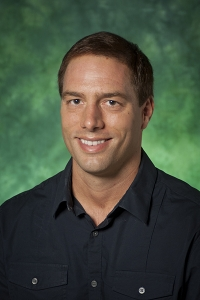#### John Krueger

Forcing Over a Free Suslin Tree

Abstract

In this talk I will discuss the concept of a free Suslin tree which was originally introduced by Jensen. It turns out that free Suslin trees can acquire a wide variety of properties by forcing. Let $$T$$ be a free Suslin tree. Then for each $$n$$ there is a proper forcing which makes $$T$$ $$n$$-free but all $$n+1$$-derived trees of $$T$$ are special. There exists a proper countably distributive forcing which adds any number of branches to $$T$$ while keeping $$T$$ rigid. And there is a proper countably distributive forcing which forces that $$T$$ carries any number of automorphisms with a strong uniformity property.#### Mariya Soskova

Stratifying classes of enumeration degrees

Abstract

Turing reducibility gives a fine-grained approach to studying mathematical objects and relationships between them. For example, Moschovakis observed that every continuous function is computable relative to some fixed Turing oracle, thus understanding relatively computable functions gives a different perspective on the study of continuous functions on the reals. This point of view belongs to effective mathematics. Turing reducibility between sets of natural numbers allows us to gauge the algorithmic content of a mathematical object, such as real number or a continuous function. However, it is not well suited to handle partial information: suppose that instead of total access to the membership in the oracle, we are only given access to the positive information. Friedberg and Rogers capture this extended model of relative computability: X is enumeration reducible to Y if there is an algorithm to enumerate X given any enumeration of Y. The induced partial order of the enumeration degrees extends the Turing degrees and carries a variety of interesting aspects. The interplay between definability, structure, and effective mathematics gives rise to a zoo of classes that informs our understating of the partial order. We take a closer look at some examples of this phenomenon.#### Assaf Shani

Models of ZF set theory with deep failure of choice

Abstract

Let $$N$$ be a model of ZF, intermediate between a ground model $$V$$ and some set-generic extension of it. Such models are usually of the form $$V(A)$$, the minimal ZF extension of $$V$$ containing $$A$$, for some set $$A$$. The first intermediate model which is not of this form is the so called Bristol model. This is an intermediate model between $$L$$ and an extension of $$L$$ by a single Cohen real.

The existence of such a model answered a question of Grigorieff from 1975. The renewed interest in the question comes from more recent work of Woodin on the HOD conjecture and the AC conjecture. In this case the problem is of particular interest when large cardinals are involved. However, the Bristol model construction requires $$L$$-like assumptions on the ground model, prohibiting the existence of large cardinals to begin with.

We give a different construction of such an "exotic intermediate model", not requiring any assumption on the ground model. Specifically: for any ground model $$V$$ there is an intermediate extension $$N$$, between $$V$$ and a Cohen-real extension, so that $$N$$ is not of the form $$V(A)$$ for any set $$A$$.

This result provides a complete solution to Grigorieff's question. Furthermore, it opens the way to investigating the following question, related to Woodin's AC conjecture: which large cardinals can exist in such a model $$N$$?

This is joint work with Yair Hayut.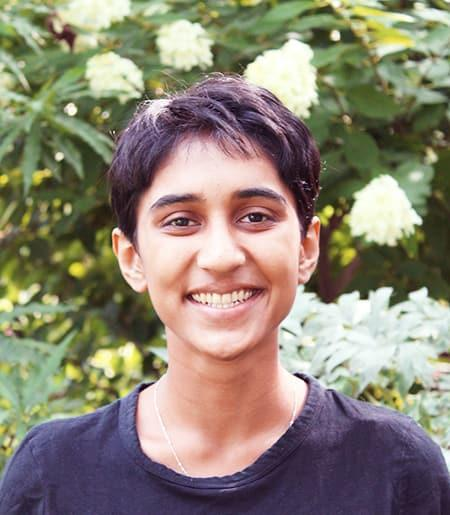#### Sumun Iyer

Generic elements in the universal Knaster continuum homeomorphism group

Abstract

A Knaster continuum is a compact, connected metrizable space which is indecomposable – in the sense that it is not the union of two non-trivial compact, connected subspaces. We will show that the Polish group $$\textrm{Homeo}^1(K)$$ of degree-one homeomorphisms of the universal Knaster continuum has a comeager conjugacy class. The result uses a particular countable chain of continuous homomorphisms of the group $$\textrm{Homeo}_+[0,1]$$ into $$\textrm{Homeo}^1(K)$$.#### Kameryn Williams

Nonstandard Methods versus Nash–Williams

Abstract

Combinatorics has long enjoyed being a site of application for nonstandard methods, using objects from ultrafilters to hyperintegers. In this talk I will discuss work from a joint project with Timothy Trujillo. We have been looking at applying nonstandard methods to the Nash–Williams partition theorem — an analogue of the infinite Ramsey theorem for certain families of finite sets of integers — and its generalizations to a broader class of Ramsey spaces.#### Discussion & HH#### Johanna Franklin

Highness for structures

Abstract

In computability theory, highness is an expression of maximal strength. We will develop the theory of highness in the context of computable structure theory. First, we will present some general results, and then we will turn to highness for particular structures such as Harrison orders and ill-founded linear orders. We will further consider these ideas in the context of other properties such as Scott completeness.#### James Cummings

Minimal non-$$\sigma$$-scattered linear orderings

Abstract

Answering a question of Baumgartner, we show that $$\diamondsuit$$ implies the existence of a minimal Countryman line. We also give the first known examples of minimal non-$$\sigma$$-scattered linear orderings of cardinality greater than $$\aleph_1$$.

This is joint work with Todd Eisworth and Justin Moore.#### Trevor Wilson

Virtual Woodin cardinals and the virtual $$n$$-Woodin hierarchy

Abstract

The notion of a virtual Woodin cardinal is obtained by weakening a certain form of the definition of Woodin cardinal to allow generic elementary embeddings in place of elementary embeddings. As usual, the virtualization is much weaker than the original: if $$0^\sharp$$ exists then every Silver indiscernible is virtually Woodin in $$L$$. Virtual Woodin cardinals are significant milestones of large cardinal strength, and for example the least virtual Woodin cardinal is the least cardinal $$\delta$$ such that every tree of cardinality $$\delta$$ without infinite branches is isomorphic to a proper subtree of itself. We give a new characterization of the virtual Woodin cardinals as Woodinized" approximations to the weakly Ramsey cardinals defined by Gitman. To be precise, we show that $$\delta$$ is virtually Woodin if and only if for every function $$f \colon \delta \to \delta$$ and every set $$A \subseteq \delta$$, there is a cardinal $$\kappa < \delta$$ closed under $$f$$, a weak $$\kappa$$-model $$M$$ containing $$f \restriction \kappa$$ and $$A \cap \kappa$$, and a weakly amenable $$M$$-ultrafilter $$U$$ on $$\kappa$$ whose ultrapower is well-founded up to $$[f \restriction \kappa]_U$$. Because the weakly Ramsey cardinals are the $$1$$-iterable cardinals, consideration of iterated ultrapowers leads us naturally to a hierarchy of virtually $$n$$-Woodin cardinals that is interleaved with the hierarchy of $$n$$-iterable cardinals. We also give a characterization of the virtually $$n$$-Woodin cardinals in terms of regressive partition relations.#### Ben Goodman

$$\Sigma_n$$-correct Forcing Axioms

Abstract

The standard method of producing a model of a forcing axiom from a supercompact cardinal in fact gives a model of an even stronger principle: that for every small name a and every $$\Sigma_2$$ formula $$\varphi$$ such that $$\varphi(a)$$ is forceable by and preserved under further forcing in our forcing class, there is a filter $$F$$ which meets a desired collection of dense sets and also interprets a such that $$\varphi(a^F)$$ already holds. I will show how to generalize this result to formulas of higher complexity by starting with slightly stronger large cardinal assumptions, producing a class of enhanced forcing axioms.#### Bartosz Wcisło

Arithmetically strong axioms for truth and satisfaction

Abstract

If we add to Peano Arithmetic (PA) a truth predicate satisfying Tarski's compositional conditions for the formulae in the base language and the full induction scheme, we can easily prove reflection over the base arithmetical language, showing that the theory containing truth predicate is not conservative over its base theory. On the other hand, it can be shown that the compositional axioms themselves are not sufficient to this goal. Tarski Boundary problem asks what assumptions have to be made about the compositional truth so that it allows to draw arithmetical conclusions going beyond PA. It turns out that the natural axioms one can assume about the truth predicate either yield conservative extensions of PA or give exactly the theory of compositional truth with the induction scheme for bounded formulae (in the expanded language), which turns out to be an unexpectedly robust theory with a number of nontrivial equivalent characterisations. In this talk, we will try to present the most important results going in this direction and possibly discuss some proof ideas.#### Discussion & HH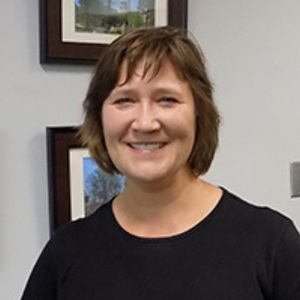#### Natasha Dobrinen

Infinite-dimensional Ramsey theory on binary relational homogeneous structures

Abstract

Generalizations of Ramsey's Theorem to colorings of infinite sets proceeds via topological considerations. The Galvin-Prikry Theorem states that Borel subsets of the Baire space are Ramsey. Silver extended this to analytic sets, and Ellentuck gave a topological characterization of Ramsey sets in terms of the property of Baire in the Vietoris topology.

We extend these theorems to several classes of countable homogeneous structures, answering a question of Kechris, Pestov, and Todorcevic. An obstruction to exact analogues of Galvin-Prikry or Ellentuck is the presence of big Ramsey degrees. We will discuss how different properties of the structures affect which analogues have been proved. Presented is work of the speaker for SDAP+ structures, and joint work with Zucker for finitely constrained binary relational FAP classes. In both works, the pigeonhole principle is achieved in ZFC by repeated applications of the forcing mechanism to find a finite object with desired properties. A feature of the work with Zucker is showing that we can weaken one of Todorcevic's four axioms guaranteeing a Ramsey space, and still achieve the same conclusion. These axioms are built on prior work of Carlson and Simpson developing topological Ramsey space theory.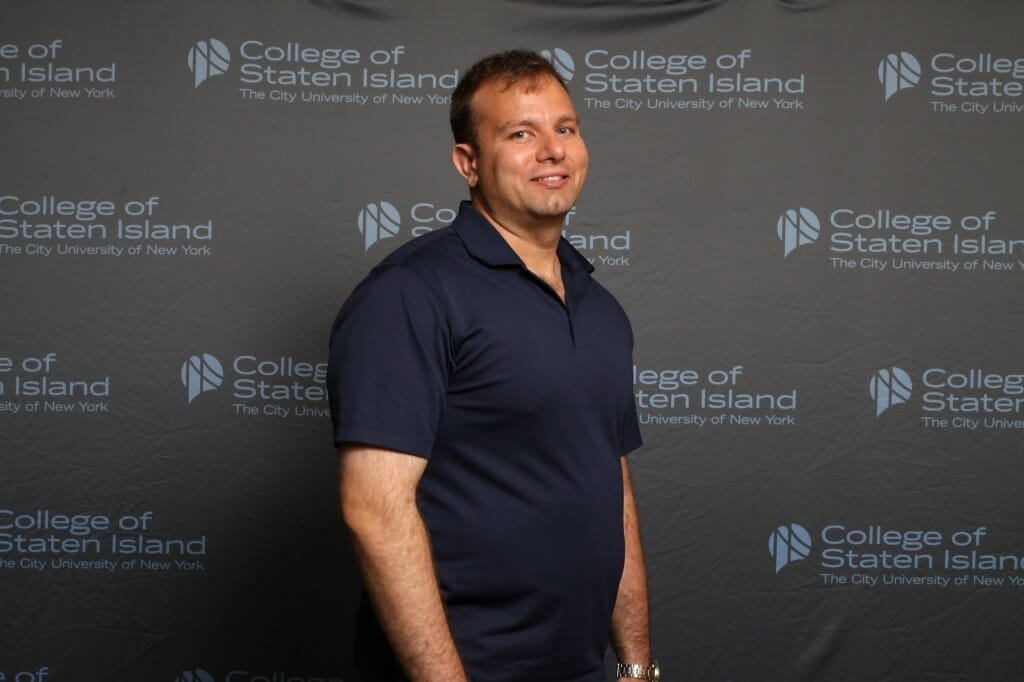#### Chris Conidis

The combinatorics of minimal prime ideals in Noetherian rings

Abstract

We will analyze the proof-theoretic complexity of the theorem from Commutative Algebra that says “every Noetherian ring has finitely many minimal prime ideals,” and relate its proof to a combinatiorial principle that asserts the existence of infinite antichains within infinite binary branching trees with infinitely many splittings.#### Gabriel Conant

Model Theoretic Results on the Functional Order Property

Abstract

In recent work on tame hypergraph regularity, Terry and Wolf introduced a higher arity analogue of stability called the “functional order property”. In this talk, I will report on joint work with Abd Aldaim and Terry, which initiates a model theoretic analysis of this notion.#### Richard Matthews

Separating Dependent Choice Principles in Set Theory without Power Set

Abstract

The scheme of dependent choice of length alpha asserts that any definable class tree without maximal nodes which is closed under sequences of length less than alpha has a branch of length alpha. In this talk we will discuss dependent choice principles over ZFC without power set. We will discuss situations where dependent choice is preserved under forcing extensions and a general framework for separating dependent choice schemes of various lengths. We will then produce a model of ZFC without power set where the collection scheme holds and there are unboundedly many cardinals, but the scheme of dependent choice of length omega fails. Most of the results in this talk are joint work with Victoria Gitman.#### Discussion & HH#### Slawek Solecki

Polish vector spaces over $$\mathbb{Q}$$

Abstract

I will present a method of producing a Polish module over an arbitrary subring of $$\mathbb{Q}$$ from an ideal of subsets of $$\mathbb{N}$$ and a sequence in $$\mathbb{N}$$. The method will be applied to construct two Polish $$\mathbb{Q}$$-vector spaces, $$U$$ and $$V$$, such that

• both $$U$$ and $$V$$ embed into $$\mathbb{R}$$ but

• $$U$$ does not embed into $$V$$ and $$V$$ does not embed into $$U$$,

where by an embedding we understand a continuous $$\mathbb{Q}$$-linear injection. This construction answers a question of Frisch and Shinko. In fact, the method produces a large number of incomparable with respect to embeddings Polish $$\mathbb{Q}$$-vector spaces.

This is joint work with Dexuan Hu.#### Andrew Brooke-Taylor

Cardinal characteristics modulo nice ideals on omega

Abstract

Cardinal characteristics of the continuum are (definitions for) cardinals that are provably uncountable and at most the cardinality of the reals, but which may be strictly less than the cardinality of the continuum in universes where the Continuum Hypothesis fails. Many of the standard cardinal characteristics of the continuum are defined in terms of a relation holding almost everywhere, where "almost everywhere" means on all but a finite set. A very natural generalisation is to take "almost everywhere" to mean on all but a member of a given ideal. I will talk about what happens when we do this, with the density $$0$$ ideal on omega as a focal example.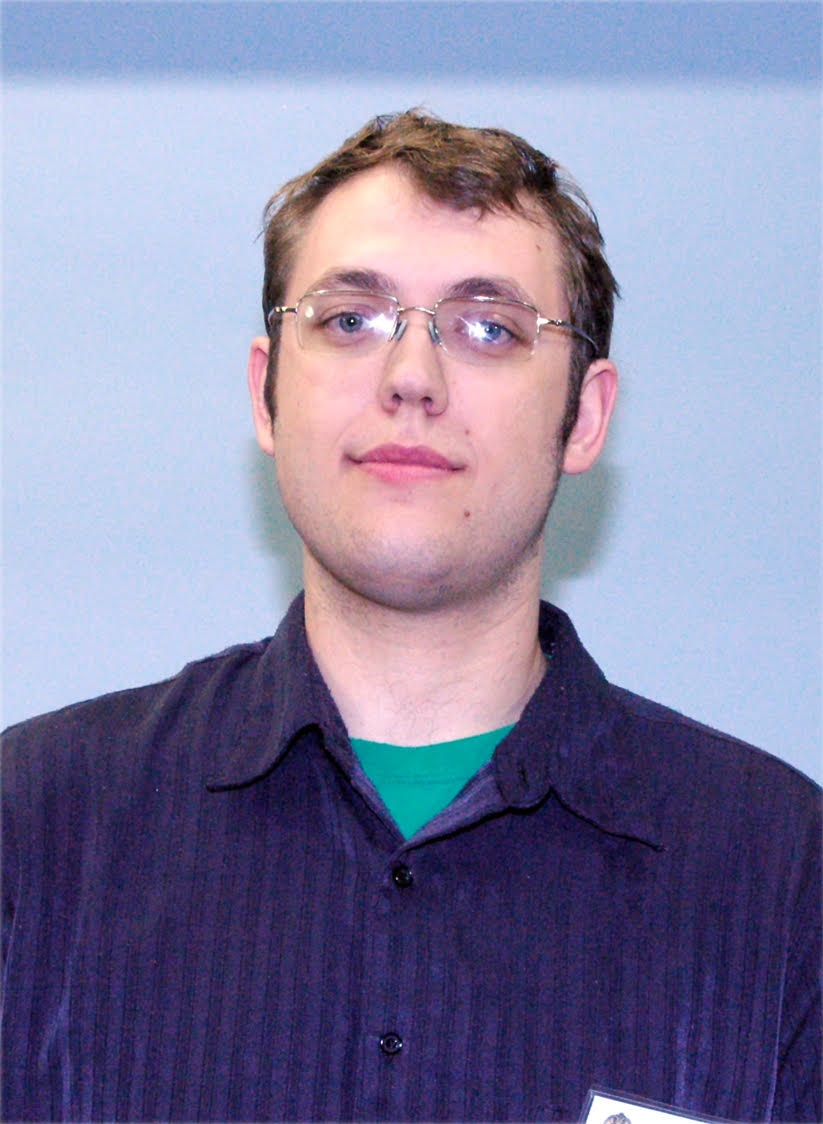#### James Hanson

Generic stability and randomizations

Abstract

Stability of a first-order theory can be characterized in terms of finite-cofinite behavior on indiscernible sequences: $$T$$ is stable if and only if for every indiscernible sequence $$I$$ and every definable set $$D$$, $$I \cap D$$ is either finite or cofinite. One important consequence of this property is that it means that any indiscernible sequence has a well-defined 'average type,' which extends uniquely to a global invariant type. It is possible for an indiscernible sequence in an unstable theory to exhibit the same property, in which case there is still a well-defined global average type of the sequence. Such types are called 'generically stable.'

It is often useful, especially in the context of NIP theories, to consider finitely additive probability measures on the Boolean algebra of definable sets, which are called Keisler measures. These form a proper generalization of types in the ordinary sense, and many questions that one can ask about types can also be asked about measures. Moreover, using continuous logic, and in particular the notion of randomizations, it is possible to literally analyze measures as types in the corresponding randomized theory $$T^R$$. The precise nature of this translation, though, can be quite subtle.

The class of 'frequency interpretation measures' is often thought of as the correct generalization of generic stability to the context of measures. Given this, one would expect that frequency interpretation measures in $$T$$ would correspond almost precisely to generically stable types in $$T^R$$, but at the moment this is less than clear. In this talk I will discuss some current research on this question.

This is joint work with Gabriel Conant and Kyle Gannon.

## Event Venue

### Rutgers, New Brunswick

Rutgers Academic Building, East wing — Room 2225

## Organizers